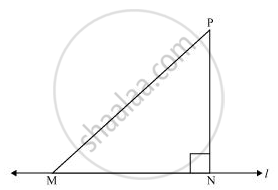# Show that of All Line Segments Drawn from a Given Point Not on It, the Perpendicular Line Segment is the Shortest. - Mathematics

Show that of all line segments drawn from a given point not on it, the perpendicular line segment is the shortest.

#### SolutionLet us take a line l and from point P (i.e., not on line l), draw two line segments PN and PM. Let PN be perpendicular to line l and PM is drawn at some other angle.

In ΔPNM,

∠N = 90º

∠P + ∠N + ∠M = 180º (Angle sum property of a triangle)

∠P + ∠M = 90º

Clearly, ∠M is an acute angle.

∴ ∠M < ∠N

⇒ PN < PM (Side opposite to the smaller angle is smaller)

Similarly, by drawing different line segments from P to l, it can be proved that PN is smaller in comparison to them.

Therefore, it can be observed that of all line segments drawn from a given point not on it, the perpendicular line segment is the shortest.

Concept: Inequalities in a Triangle
Is there an error in this question or solution?

#### APPEARS IN

NCERT Class 9 Maths
Chapter 7 Triangles
Exercise 7.4 | Q 6 | Page 133